• 解耦算法
2021-04-20 07:14:04

摘要：本次设计是针对温度与水位的控制，在一套系统中同时解决多个控制变量之间互相的干扰，又使多个变量同时处于规定的要求值内。因此在本次毕业设计中设计了基于解耦算法的三容水箱其中的温度与液位解耦系统实验,其中用到磁力泵，变频器作为控制器，温度与水位则是被控变量，有效控制冷水的进水速度与热水的进水速度这两个控制变量。建立了三容水箱系统的数学模型,在matlab中进行了仿真。并以此为基础利用解耦原理设计了解耦控制器,并对实际的三容水箱中的上水箱的温度与液位进行了解耦控制,最后通过实验得到解耦曲线图，实验结论表明解耦控制能够有效地控制两种互相干扰的变量。57461

毕业论文关键词: 三容水箱，液位控制，解耦算法

Water tank control system based on decoupling algorithm

Abstract：The design is to control the temperature and water level, in a set of systems to solve the multiple control variables at the same time the interference between each other, so that the number of variables at the same time within the required value. So in this graduation design is designed based on the decoupling algorithm of three tank water the temperature and level decoupling system experiment, which uses magnetic pump, frequency converter as controller, temperature and water level is controlled variable, the effective control of the cold water inlet velocity and the hot water inlet velocity of the two control variables. The mathematical model of the three tank system is established, and the simulation is carried out in MATLAB. And thus is based on using decoupling control theory is applied to design a decoupling controller, and decoupling control of actual three tank water tank of the water tank temperature and liquid level, finally, decoupling curves are obtained through the experiment, the experimental results show that decoupling control can effectively control the two interference variables.

Key words：Three tank water tank, liquid level control, decoupling algorithm

目录

1.绪论1

1.1课题的提出与意义1

1.2解耦控制的发展概况与未来的发展趋势1

1.3论文的主要研究内容4

2.基于解耦算法的水箱控制系统设计以及仿真5

2.2解耦控制系统6

2.3控制系统设计9

2.3.1确定控制器参数9

2.3.2选择参数10

2.4仿真调试12

3.基于解耦算法的水箱控制系统实验13

3.1 实验平台简介13

3.2 实验操作15

3.3 实验结果及比较17

4.总结与展望19

致谢21

参考文献22

1.绪论

1.1 课题的提出与意义

随着科学技术的发展,工业生产过程中的自动控制已经成为一门重要的内容，在日常生活及工业控制方面得到了广泛的应用，然而在设计控制系统时并不简单，首先要对系统要求进行分析并且建模，通过对水位、温度控制的设计，制作、了解信息采集测试、校准、控制及通信的全过程，提高在电子工程设计和实际操作方面的运用能力。

培养研发能力，通过对工业生产的控制设计，掌握在给定条件和要求的情况下，如何达到经济实用的方法，巧妙合理地去设计工程系统中的某一部分程序，并将其运动到实际工业体系中。

针对要求做的模型,做相应的分析,继而设计相应的控制系统,最后对系统的稳定性等各项性能进行分析。

1.2解耦控制的发展概况与未来的发展趋势

在人们生活中以及工业生产过程中常常会有类似水箱的控制问题，常见的例如饮料的制作过程中的进出量、食品加工，居民生活用水的供应，溶液在质量要求下的过滤，化工生产等类似行业的生产加工过程，往往要使用蓄液池。蓄液池中的液位与温度需要维持合适的可控量内，太满容易溢出造成浪费，太少就无法满足需求，温度太高对容器会有损伤，温度太低不能满足用户要求。 matlab基于解耦算法的水箱控制系统设计:http://www.lwfree.com/zidonghua/lunwen_62125.html

更多相关内容
• 预紧式并联六维加速度传感器的解耦算法研究.pdf,针对六维加速度传感器的解耦难度与构型复杂度存在矛盾的现状，给出了12支链预紧式并联六维加速度传感器的设计方案，并在四维位形空间内构建了解耦算法。通过重组输入...
• 基于径向基函数神经网络的压电式六维力传感器解耦算法.pdf
• 本文提出了一种基于全变差模型的超分辨率复原快速解耦算法.利用半二次正则化思想,提出了一个新的解耦TV(Total variation)模型.利用交替最小化方法和线性空间不变模糊的性质将上采样融合、去模糊和去噪分步进行.算法...
• 针对传感器输出电压正负方向拟合函数不同提出了一种基于耦合误差和分段拟合建模的六维力传感器解耦算法。用十字梁结构的传感器进行标定试验，将得到的标定数据应用于解耦算法中。通过标定数据验证了基于耦合误差和...
•研究论文
• 两热传导方程耦合问题的解耦算法研究_闫伟(1).caj
• 给出一种新的用于转子磁场直接定向矢量控制系统的解耦电压计算方法，通过计算机仿真分析和实验，对其转矩响应能力等控制性能进行研究并给出结论，仿真采用Matlab6.1软件。
• 在电机控制、逆变器控制、PWM整流器等领域中，常使用电压前馈解耦方法用于电流内环的控制。但是之前本人不理解电压前馈解耦是如何实现的，认为这张图1里idiq还是有耦合实现啊。后面仔细看公式之后，算是对电压前馈...

目录

0 引言

1 理论分析

2 结论

# 0 引言

在电机控制、逆变器控制、PWM整流器等领域中，常使用电压前馈解耦方法用于电流内环的控制。但是之前本人不理解电压前馈解耦是如何实现的，认为这张图1里id iq还是有耦合项啊。后面仔细看公式之后，算是对电压前馈解耦方法理解透彻了。

# 1 理论分析

以三相逆变器（整流器）为例，三相电流经ABC->d-q变换后得到如下模型：

显然，id与iq在模型上互相有关，即相互耦合，为了使d-q轴的电流环设计完全一样，我们需要对它进行解耦合。

① 把式（1）左右移相，得式（2）

② 电流调节器采用PI控制器，则式（2）变换为式（3）

③ 把式（3）带入式（2）中，得式（4）

④ 显然，与式（1）相比，式（4）中id与iq实现了完全解耦，并且完全对称。所以d-q轴电流环可以独立设计，并且完全一样。

# 2 结论

经过节1的理论推导，算是透彻理解了前馈解耦算法的原理哦，前馈解耦的策略就是图1所示

展开全文• 文章目录前言一、磁流体的无条件能量稳定解耦算法二、两相磁流体的无条件能量稳定解耦算法总结 前言 磁流体问题是一个多物理场耦合的问题。如何设计有效的无条件能量稳定解耦算法一直是研究的热点。 一、磁流体的...

# 前言

磁流体问题是一个多物理场耦合的问题。如何设计有效的无条件能量稳定的解耦算法一直是研究的热点。

# 一、磁流体的无条件能量稳定的解耦算法

磁流体方程是由NS方程和Maxwell方程耦合得到的。由于Maxwell方程的形式比较多，磁流体模型也多种多样。下面主要讲解以磁场 B B 为变量的磁流体方程组的解耦算法，稍后将其推广到更一般的磁流体模型和其他具有类似性质的模型。

磁流体方程为边界条件为初始条件为我们知道这个磁流体方程满足如下的能量守恒律其中总的能量定义为其解耦算法如下：

1. 求解磁方程，
B n + 1 − B n δ t + η ∇ × ∇ × B n + 1 − ∇ × ( u ⋆ n × B n ) = 0 ∇ ⋅ B = 0 B n + 1 × n ∣ ∂ Ω = 0 \frac{\boldsymbol{B}^{n+1}-\boldsymbol{B}^{n}}{\delta \boldsymbol{t}}+\eta \nabla \times \nabla \times \boldsymbol{B}^{n+1}-\nabla \times\left(\boldsymbol{u}_{\star}^{n} \times \boldsymbol{B}^{n}\right)=0 \\ \nabla \cdot \boldsymbol{B} =0\\ \boldsymbol{B}^{n+1} \times\left.\boldsymbol{n}\right|_{\partial \Omega}=0
其中 u ⋆ n = u n − s δ t B n × ∇ × B n + 1 \boldsymbol{u}_{\star}^{n}=\boldsymbol{u}^{n}-s \delta t \boldsymbol{B}^{n} \times \nabla \times \boldsymbol{B}^{n+1} 为传输速度。它的引入对无条件能量稳定的证明至关重要。

2. 求解NS方程，
u n + 1 − u n δ t + ( u n ⋅ ∇ ) u n + 1 − v Δ u n + 1 + ∇ p n + s B n × ∇ × B n + 1 = 0 ∇ ⋅ u = 0 u n + 1 ∣ ∂ Ω = 0 \frac{{\boldsymbol{u}}^{n+1}-\boldsymbol{u}^{n}}{\delta t}+\left(\boldsymbol{u}^{n} \cdot \nabla\right) {\boldsymbol{u}}^{n+1}-v \Delta {\boldsymbol{u}}^{n+1}+\nabla p^{n}+ s\boldsymbol{B}^{n} \times \nabla \times \boldsymbol{B}^{n+1}=0\\ \nabla \cdot \boldsymbol{u} =0\\ \left.{\boldsymbol{u}}^{n+1}\right|_{\partial \Omega}=0

我们可以发现得到的格式为线性解耦，并且很容易得到无条件稳定性的证明。这里并没有将速度和压力解耦，是为了保证流体这一部分的精度。当然，也可以将其解耦。格式构造的关键是引入传输速度 u ⋆ n \boldsymbol{u}_{\star}^{n} , 可以看到 u ⋆ n \boldsymbol{u}_{\star}^{n} 的方程中含有洛伦兹力项。粗略地讲，这个速度刻画了洛伦兹力对流体系统的影响。有了这一观察后，很容易将这一格式推广到其他磁流体模型中。下面一节将其推广到两相流磁流体中。

总结一下，利用洛伦兹力引入传导速度然后将磁方程和流体方程解耦。当然，也可以先求解NS方程，再求解Maxwell方程。这时候，需要用诱导电流引入传输磁场从而构造解耦的格式，格式是类似。目前，我正在将这种方法做一个统一的框架。

## 参考文献

1.G. D. Z. , X. H. , & X. Y. . (0). Fully decoupled, linear and unconditionally energy stable time discretization scheme for solving the magneto-hydrodynamic equations. Journal of Computational and Applied Mathematics, 369.
2. Zhang, G. D. , He, X. M. , & Yang, X. F. . (2019). A decoupled, linear and unconditionally energy stable scheme with finite element discretizations for magneto-hydrodynamic equations. Journal of entific Computing, 81(3), 1678-1711.
3. Santiago Badia, Ramon Planas, & Juan Vicente Gutiérrez-Santacreu. (2013). Unconditionally stable operator splitting algorithms for the incompressible magnetohydrodynamics system discretized by a stabilized finite element formulation based on projections. International Journal for Numerical Methods in Engineering.
4. Xiaodi Zhang. Decoupled, linear and unconditionally energy stable time discretization scheme for solving the inductionless magnetohydrodynamic equations, submited.

# 二、两相磁流体的无条件能量稳定的解耦算法

求解磁流体两相流的一种有效的方法是相场方法，其模型为将MHD方程和CH方程耦合起来。同样以以磁场 B B 为变量，这里讲解一种将Maxwelll方程、NS方程和CH方程解耦的无条件能量稳定的算法。

其方程形式为利用之前的思路，注意到这里有三个系统，因此可以按照顺序不同给出6种不同的算法。这里仅以一种为例，

1. 求解相场方程，
ϕ n + 1 − ϕ n δ t + ∇ ⋅ ( u ∗ n ϕ n ) − γ Δ w n + 1 = 0 w n + 1 + 1 ϵ 2 ( Δ ϕ n + 1 − f c ( ϕ n + 1 ) + f c ( ϕ n ) ) = 0 ϕ n + 1 ∣ ∂ n = 0 , ∂ n w n + 1 ∣ ∂ n = 0. \frac{\phi^{n+1}-\phi^{n}}{\delta t}+\nabla \cdot\left(u_{*}^{n} \phi^{n}\right)-\gamma \Delta w^{n+1}=0 \\ w^{n+1}+\frac{1}{\epsilon^2}\left(\Delta \phi^{n+1}-f_{c}\left(\phi^{n+1}\right)+f_{c}\left(\phi^{n}\right)\right)=0 \\ \left. \phi^{n+1}\right|_{\partial n}=0,\left.\partial_{n} w^{n+1}\right|_{\partial n}=0.
其中 u ⋆ n = u n − λ δ t ϕ n ∇ w n + 1 \boldsymbol{u}_{\star}^{n}=u^{n}-\lambda\delta t \phi^{n} \nabla w^{n+1} 为相场传输速度。
2. 求解磁方程，
B n + 1 − B n δ t + 1 μ ∇ × ( 1 σ ( ϕ n + 1 ) ∇ × B n + 1 ) − ∇ × ( u ⋆ ⋆ n × B n ) = 0 ∇ ⋅ B = 0 B n + 1 × n ∣ ∂ Ω = 0 \frac{\boldsymbol{B}^{n+1}-\boldsymbol{B}^{n}}{\delta \boldsymbol{t}}+\frac{1}{\mu} \nabla \times (\frac{1}{\sigma(\phi^{n+1})}\nabla \times \boldsymbol{B}^{n+1})-\nabla \times\left(\boldsymbol{u}_{\star\star}^{n} \times \boldsymbol{B}^{n}\right)=0 \\ \nabla \cdot \boldsymbol{B} =0 \\ \boldsymbol{B}^{n+1} \times\left.\boldsymbol{n}\right|_{\partial \Omega}=0
其中 u ⋆ ⋆ n = u ⋆ n − s δ t B n × ∇ × B n + 1 \boldsymbol{u}_{\star\star}^{n}=\boldsymbol{u}_{\star}^{n}-s \delta t \boldsymbol{B}^{n} \times \nabla \times \boldsymbol{B}^{n+1} 为磁场传输速度。
3. 求解NS方程，
ρ （ u n + 1 − u n δ t + ( u n ⋅ ∇ ) u n + 1 − 2 d i v ( η ( ϕ n + 1 ) D ( u n + 1 ) ) + ∇ p n + 1 μ B n × ∇ × B n + 1 + λ ϕ n ∇ w n + 1 = 0 , \rho（\frac{{\boldsymbol{u}}^{n+1}-\boldsymbol{u}^{n}}{\delta t}+\left(\boldsymbol{u}^{n} \cdot \nabla\right) {\boldsymbol{u}}^{n+1}-2 \rm{div} (\eta(\phi^{n+1})D({\boldsymbol{u}}^{n+1}))+\nabla p^{n}+ \frac{1}{\mu}\boldsymbol{B}^{n} \times \nabla \times \boldsymbol{B}^{n+1}+\lambda\phi^{n} \nabla w^{n+1}=0,
∇ ⋅ u = 0 u n + 1 ∣ ∂ Ω = 0 \nabla \cdot \boldsymbol{u} =0 \\ \left.{\boldsymbol{u}}^{n+1}\right|_{\partial \Omega}=0

我们很容易得到能量无条件稳定的证明。这种解耦的思路在相场两相流相关的文章经常见到。这里将其应用到两相磁流体中。显然，这种方法的应用比较广泛。完全可以作为一种框架去使用。感兴趣的读者，可以将其应用到铁磁流体中，构造格式的方法是一样的。

最后，这种构造格式的方法当然也有缺点。首先，当把耦合系统解耦后，解耦的单个系统的解的存在唯一性没法得到直接的保证，有时候需要比较小的步长。其次，这种解耦格式仅限于一阶，更高阶的格式，还没有在文献中见到。

## 参考文献

1. Guillén-González, F, & Tierra, G. . (2018). Unconditionally energy stable numerical schemes for phase-field vesicle membrane model. Journal of Computational Physics, 354, 67-85.
2. (2016). A decoupled energy stable scheme for a hydrodynamic phase-field model of mixtures of nematic liquid crystals and viscous fluids. Journal of Computational Physics, 305, 539-556.
3. Shen, J. , & Yang, X. . (2015). Decoupled, energy stable schemes for phase-field models of two-phase incompressible flows. Siam Journal on Numerical Analysis, 53(1), 279-296.
展开全文经验分享
• 前馈补偿解耦法设计一已知的两输入、两输出有耦合被控对象的解耦控制系统，并完成它的混合仿真，对无耦合系统、有耦合而未解耦系统以及有耦合且采用解耦控制系统的控制作比较研究
• 分析了运动解耦机理，提出了只有结构解耦才能方便地做到运动解耦，给出了结构解耦的条件：所有回转轴线交于一点，前面的转动使得有关回转轴线的位姿发生变化，后面的转动按照已经发生变化的回转轴线转动，即保证杆的...
• 感应电机矢量控制解耦算法的研究,希望对您有帮助．
• 这是PID神经元网络解耦控制算法的MATLAB源代码，请大家多多参考！！！
• PID神经元网络解耦控制算法_多变量系统控制，PID神经元是人工神经网络的类型PID
• 针对一类多变量离散时间非线性动态系统, 分别设计线性鲁棒自适应解耦控制律和神经网络非线性自适应解耦控制律.根据指定的性能指标,通过它们之间的切换对系统进行控制.理论分析和仿真结果表明,该控制策略不但可以保证...
• 对由大行程变形镜和高空间频率变形镜组成的双变形镜自适应光学系统中的像差解耦原理和限定像差校正算法做了理论分析。认为在高空间频率变形镜的斜率响应矩阵中加入限定像差向量,根据直接斜率法分别计算出两个变形镜...
• 提出一种求解最优潮流(OPF)问题的新算法--解耦半光滑牛顿型算法....解耦算法可达到加快计算速度、 提高计算效率的目的.IEEE多个算例的数值实验以及与其他方法的比较均显示了新算法具有良好的计算效果.</p>
• 对日益大型化、复杂化的隔振系统进行主动控制，采用多误差的最小均方差算法(LMS)...仿真和试验结果表明，通过调整收敛系数和控制代价加权因子，主动隔振系统采用简单易行的解耦算法对线谱振动进行控制是行之有效的。
• 资源名：PID神经元网络解耦控制算法_多变量系统控制_matlab 资源类型：matlab项目全套源码 源码说明： 全部项目源码都是经过测试校正后百分百成功运行的，如果您下载后不能运行可联系我进行指导或者更换。 适合人群...
• PID神经元网络解耦控制，多变量控制 matlab实现
• 提出一种求解最优潮流（OPF）问题的新算法——解耦半光滑牛顿型算法。...解耦算法可达到加快计算速度、提高计算效率的目的。IEEE多个算例的数值实验以及与其他方法的比较均显示了新算法具有良好的计算效果。
• 基于麻雀搜索的PID神经网络解耦控制算法 文章目录基于麻雀搜索的PID神经网络解耦控制算法1.PID神经元网络结构2.控制律计算3.权值修正4.控制对象5.模型建立6.基于麻雀搜索的PID神经网络解耦控制算法7.算法结果：8....

# 基于麻雀搜索的PID神经网络解耦控制算法

摘要：PID 神经元网络采用的学习算法是梯度学习法，初始权值随机得到，权值在学习过程中可能陷入局部最优值。采用麻雀搜索算法优化神经元网络初始权值。

## 1.PID神经元网络结构

PID 神经元网络从结构上可以分为输入层、隐含层和输出层三层， n 个控制量的 PID 神经 元网络包含 n 个并列的相同子网络 ， 各子网络间既相互独立 ， 又通过网络连接权值相互联系。 每个子网络的输入层有两个神经元，分别接收控制量的目标值和当前值。每个子网络的隐含 层 由比例元、积分元和微分元构成，分别对应着 PID 控制器中的比例控制、积分控制和微分控制。 PID 神经元网络按被控系统控制量的个数可以分为控制单变量系统的单控制量神经元网 络和控制多变量系统的多控制量神经元网络。其中单控制量神经元网络是PID 神经元网络的基本形式，多控制量神经元网络可以看成是多个单控制量神经元网络的组合形式。单控制量神经元网络的拓扑结构如图1所示。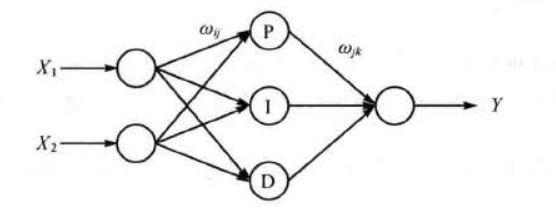图1.单控制量神经元网络的拓扑结构

图 1中 X 1 X_1 是控制量的控制目标 ， X 2 X_2 是控制量当前值 ， Y Y 是神经元网络计算得到的控制律， w i j w_{ij} w j k w_{jk} 是网络权值，从中可以看到单控制量神经元网络是一个三层前向神经元网络，网络结构为 2-3- 1 ，隐含层包含比例元、积分元和微分元三个神经元。多控制量神经元网络可 以看成多个单控制量网络的并联连接，多控制量神经元网络拓扑结构如图 2 所示。图中， $X_{11} , X_{21} ， … ， X_{n1}$是控制量的控制目标 ； X 12 , X 22 ， … ， X n 2 X_{12} , X_{22} ， …， X_{n2} 是控制量的当前值； Y 1 , . . , Y n Y_1,..,Y_n 是多控制量神经元网络计算得到的控制律 ； w i j w_{ij} w j k w_{jk} 是网络权值。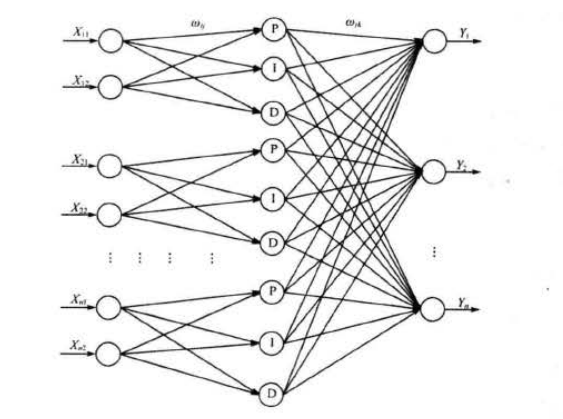图2.多控制量神经元拓扑网络

## 2.控制律计算

PID 神经元网络分为输入层、隐含层和输出层，网络输入量为控制量当前值和控制目标， 输出蛊为控制律，各层输入输出计算公式如下 。

(1) 输入层

输入层中包含2n个神经元，输出数据 x s i x_{si} 等于输入数据 X s i X_{si} 计算公式为:
x s i ( k ) = X s i ( k ) (1) x_{si}(k) = X_{si}(k) \tag{1}
(2) 隐含层

隐含层有 3n 个神经元，包括 n 个比例神经元、n 个积分神经元和 n 个微分神经元。这些神经元的输入值相同，计算公式为 ：
n e t i j ( k ) = ∑ i = 1 2 w i j x s i ( k ) , j = 1 , 2 , 3 (2) net_{ij}(k) = \sum_{i =1}^2 w_{ij}x_{si}(k),j = 1,2,3 \tag{2}
隐含层各神经元输出的计算公式如下：

比例神经元： u s 1 ( k ) = n e t s 1 ( k ) u_{s1}(k) = net_{s1}(k)

积分神经元： u s 2 ( k ) = n e t s 2 ( k ) + u s 2 ( k − 1 ) u_{s2}(k) = net_{s2}(k) + u_{s2}(k-1)

微分神经元： u s 3 ( k ) = n e t s 3 ( k ) − n e t s 3 ( k − 1 ) u_{s3}(k) = net_{s3}(k) - net_{s3}(k-1)

式中 ，s 为并联子网络的序号; j为子网络中隐含层神经元序号, x s i ( k ) x_{si}(k) 为各子网络输入层神经元输出值 ； w i j w_{ij} 为各子网络输入层至隐含层的连接权重值 。

(3)输出层

输出层有 n 个神经元 ，构成 n 维输出量，输出层的输出为隐含层全部神经元的输出值加权和，计算公式如下：
y h ( k ) = ∑ s = 1 n ∑ j = 1 3 w j k u s j ( k ) (3) y_h(k) = \sum_{s=1}^{n} \sum_{j=1}^3 w_{jk}u_{sj}(k) \tag{3}
式中，h 为输出层神经元序号；s为子网的序号；j为子网的隐含层神经元序号 ； u s j ( k ) u_{sj}(k) 为隐含层各神经元输出值 ； w j k w_{jk} 为隐含层至输出层的连接权重值。

## 3.权值修正

PID 神经元网络在控制的过程中根据控制量误差按照梯度修正法修正权值，使得控制量不断接近控制目标值 ，权值修正的过程如下 。

误差计算公式如下：
J = ∑ E = ∑ k = 1 n [ y h ( k ) − r ( k ) ] 2 (4) J = \sum E = \sum_{k=1}^n[y_h(k) - r(k)]^2 \tag{4}
式中，n为输出节点个数， y h ( k ) y_h(k) 为预测输出； r ( k ) r(k) 为控制目标。

PID神经元网络权值修正公式如下：

a.输出层到隐含层：
w j k ( k + 1 ) = w j k ( k ) − η δ J δ w j k (5) w_{jk}(k+1) = w_{jk}(k) - \eta\frac{\delta J}{ \delta w_{jk}} \tag{5}
b.输入层到输出层
w i j ( k + 1 ) = w i j ( k ) − η δ J δ w i j (6) w_{ij}(k+1) = w_{ij}(k) - \eta\frac{\delta J}{ \delta w_{ij}} \tag{6}
其中 η \eta 为学习速率。

## 4.控制对象

PID 神经元网络的控制对象是一个 3 输入 3 输出的复杂耦合系统，系统的传递函数如下：
{ y 1 ( k ) = 0.4 ∗ y 1 ( k − 1 ) + u 1 ( k − 1 ) / [ 1 + u 1 ( k − 1 ) 2 ] + 0.2 u 1 ( k − 1 ) 3 + 0.5 u 2 ( k − 1 ) + 0.3 y 2 ( k − 1 ) y 2 ( k ) = 0.2 ∗ y 2 ( k − 1 ) + u 2 ( k − 1 ) / [ 1 + u 2 ( k − 1 ) 2 ] + 0.4 u 2 ( k − 1 ) 3 + 0.2 u 1 ( k − 1 ) + 0.3 y 3 ( k − 1 ) y 3 ( k ) = 0.3 ∗ y 3 ( k − 1 ) + u 3 ( k − 1 ) / [ 1 + u 3 ( k − 1 ) 2 ] + 0.4 u 3 ( k − 1 ) 3 + 0.4 u 2 ( k − 1 ) + 0.3 y 1 ( k − 1 ) (7) \begin{cases} y_1(k) = 0.4*y_1(k-1)+u_1(k-1)/[1+u_1(k-1)^2] + 0.2u_1(k-1)^3+0.5u_2(k-1)+0.3y_2(k-1)\\ y_2(k) = 0.2*y_2(k-1)+u_2(k-1)/[1+u_2(k-1)^2] + 0.4u_2(k-1)^3+0.2u_1(k-1)+0.3y_3(k-1)\\ y_3(k) = 0.3*y_3(k-1)+u_3(k-1)/[1+u_3(k-1)^2] + 0.4u_3(k-1)^3+0.4u_2(k-1)+0.3y_1(k-1)\\ \end{cases}\tag{7}
从式7中可以看出该系统的控制量相互搞合，用 一般的控制方法难以取得理想的控制效果。

## 5.模型建立

PID 神经元网络控制器和被控系统构成的闭环控制系统如图 3所示：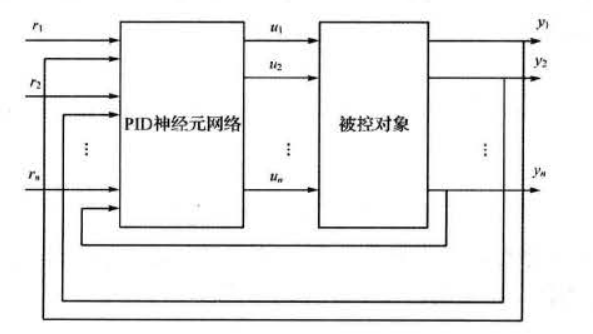图3.PID神经元网络闭环控制系统

图中, r 1 , . . . , r n r_1,...,r_n 是控制量控制目标 ， u 1 , . . . , u n u_1,...,u_n 为控制器控制律， y 1 , . . . , y n y_1,...,y_n 为控制量当前值。对于本案例来说，由于被控对象有三个控制量，所以选择包含三个单神经元网络组成的多神经元网络作为系统控制器． 网络权值随机初始化，控制量初始值为[0,0,0]，目标为[0.7,0.4,0.6],控制时间间隔为0.001s。

## 6.基于麻雀搜索的PID神经网络解耦控制算法

麻雀搜索算法的具体原理参考博客：https://blog.csdn.net/u011835903/article/details/108830958。

PID 神经元网络采用的学习算法是梯度学习法，初始权值随机得到，权值在学习过程中可能陷入局部最优值。采用麻雀搜索算法优化神经元网络初始权值。适应度函数即为控制误差和。

麻雀算法参数设置如下：

pop=50; % 种群数量
Max_iteration=40; % 设定最大迭代次数
dim = 3*6 + 3*9;% 权值维度
lb = -0.3;%上边界
ub = 0.3;%下边界
fobj = @(x) fun(x);%适应度函数



## 7.算法结果：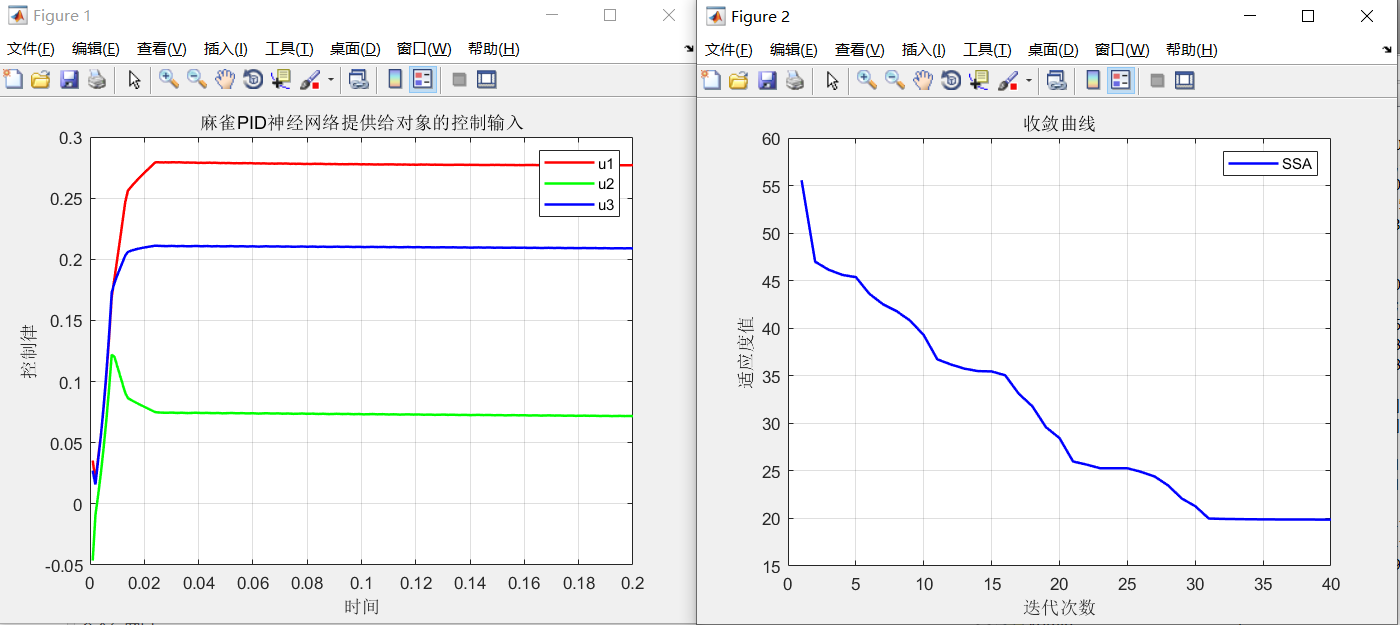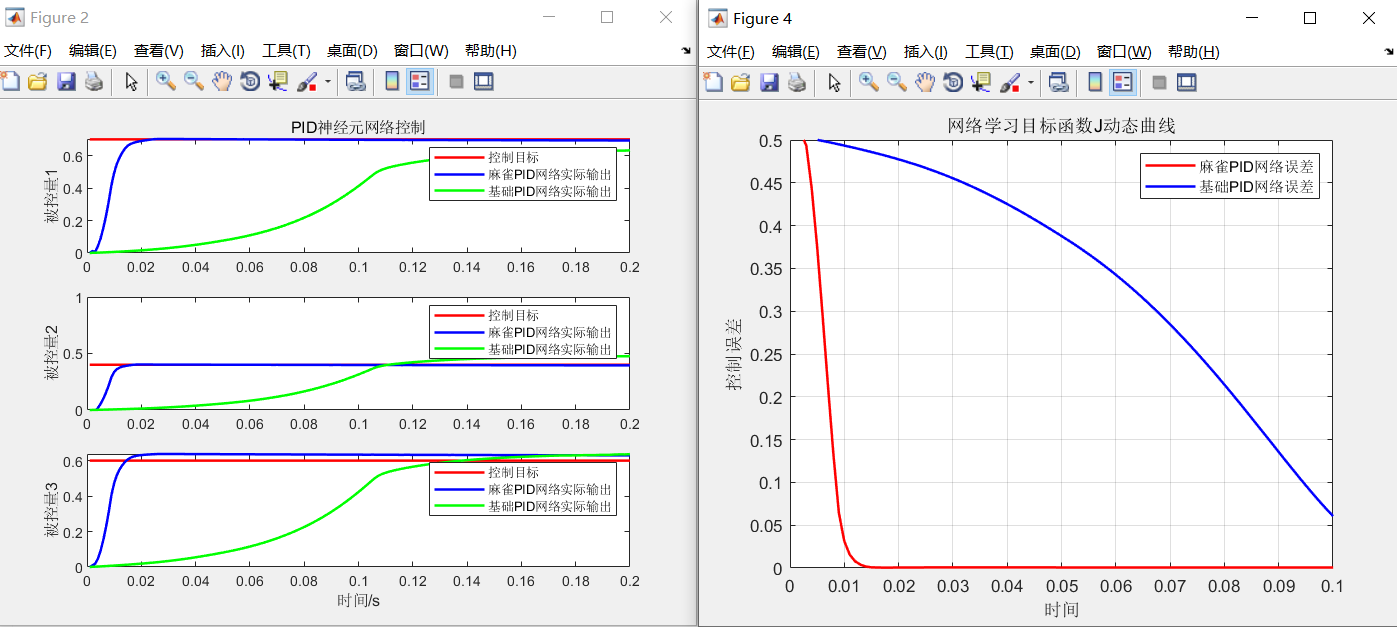从结果上来看，改进后的麻雀算法收敛速度快，控制精度明显高于基础的PID网络，且控制时间明显优于基础PID网络误差。

## 8.参考文献：

书籍《MATLAB神经网络43个案例分析》，上文基本原理均摘自该书籍。

## 9.Matlab代码

个人资料介绍

展开全文深度学习 神经网络 人工智能...